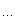﻿ C++中的强制类型转换 - 王牌软件

# C++中的强制类型转换

const_cast，reinterpret_cast，static_cast，dynamic_cast等等。

1）static_cast<T*>(a)class B {};class D : public B {};void f(B* pb, D* pd){D* pd2 = static_cast<D*>(pb);        // 不安全, pb可能只是B的指针B* pb2 = static_cast<B*>(pd);        // 安全的2）dynamic_cast<T*>(a)class A {};class B {};void f(){A* pa = new A;B* pb = new B;void* pv = dynamic_cast<A*>(pa);// pv 现在指向了一个类型为A的对象pv = dynamic_cast<B*>(pb);// pv 现在指向了一个类型为B的对象}3）const_cast<T*>(a)class A {};void f(){const A *pa = new A;//const对象A *pb;//非const对象//pb = pa; // 这里将出错，不能将const对象指针赋值给非const对象pb = const_cast<A*>(pa); // 现在OK了..   }4）reinterpret_cast<T*>(a)class A {};class B {};void f(){A* pa = new A;void* pv = reinterpret_cast<A*>(pa);// pv 现在指向了一个类型为B的对象，这可能是不安全的}0
﻿
Ɣ回顶部